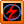### 1Ohms Law calculator 2

These is the updated version of calculator of  the voltage , current, power and resistance of a linear circuit.
These formulas are derive from the Ohms law pie chart. The formulas are as  follows:

Current (I)     = Voltage / Resistance
= Power / Voltage
= square root of  Power / Resistance
Resistance (Ω) = Power/Current squared
= Voltage squared / Power
= Voltage / Current
Power (W)    = Voltage * Current
= Current squared * Resistance
=Voltage squared / Resistance
Voltage (V)  = Current * Resistance
=  Power / Current
= square root of Power * Resistance

How to use:
1. Input two known values such as voltage and current, and etch.
2. Choose the units on both inputs and outputs
3. Click calculate button to solve
4. Click Clear button if you want to try again and return to step 1Ohm's Law pie chart

 Voltage V µV mV kV Resistance Ω µΩ mΩ kΩ MΩ Current A µA mA kA Power W µW mW kW1.